Courses

# Test: Money - 3

## 20 Questions MCQ Test Mathematics (Maths) for Class 4 | Test: Money - 3

Description
This mock test of Test: Money - 3 for Class 4 helps you for every Class 4 entrance exam. This contains 20 Multiple Choice Questions for Class 4 Test: Money - 3 (mcq) to study with solutions a complete question bank. The solved questions answers in this Test: Money - 3 quiz give you a good mix of easy questions and tough questions. Class 4 students definitely take this Test: Money - 3 exercise for a better result in the exam. You can find other Test: Money - 3 extra questions, long questions & short questions for Class 4 on EduRev as well by searching above.
QUESTION: 1

Solution:
QUESTION: 2

Solution:
QUESTION: 3

### Codi buys a bat for Rs.245, a ball for Rs. 30, If he had total Rs.3000 with him, how much money left with him?

Solution:
QUESTION: 4

There are 6665 students in a school. Each student contributed Rs. 53 for a society welfare programme. How much many the students contributed together?

Solution:
QUESTION: 5

Price of 3 kg apple is twice of the price of 1 kg mango. Robert buys 4 kg apple 4 kg mango, if  of mango is Rs. 75 /kg, find the amount of money Robert has to pay.

Solution:
QUESTION: 6

Sharad bought a pencil box for Rs. 35.75 and a notebook, for Rs. 22, 10. Find the total amount spent.

Solution:
QUESTION: 7

Nirmala bought a T-shirt for Rs.150, a pair of shoes for Rs.269.50 and a suit for Rs. 650.75. If she gave three 500-rupee notes, how much did she get back?

Solution:
QUESTION: 8

Sita has Rs.100 with her. She bought a bucket and had Rs.3.10 left with How much did the bucket cost?

Solution:
QUESTION: 9

Sushil bought 8 dozen balls at the rate of Rs.88, 20 per dozen. How much did he pay to the seller?

Solution:
QUESTION: 10

Paul was going on a sponsored walk. The walk was 8 km. He collected Rs.80 from his dad, Rs.2.70 from his mom and Rs.40 from his sister How much did he collect altogether?

Solution:
QUESTION: 11

Directions: for questions: See the table blow and answer the following questions: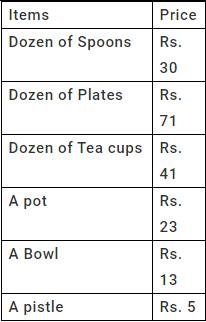Q. Which item is the most expensive?

Solution:
QUESTION: 12

Directions: for questions: See the table blow and answer the following questions: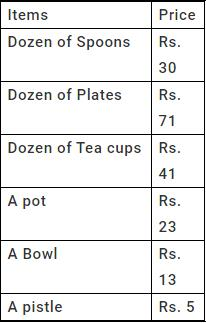Q. Which item is the least expensive?

Solution:
QUESTION: 13

Directions: for questions: See the table blow and answer the following questions: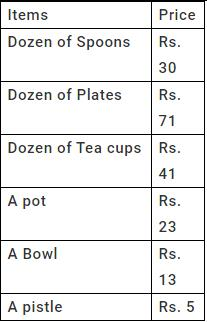Q. How much is the dozen of plates more expensive than a dozen of tea cups?

Solution:
QUESTION: 14

Directions: for questions: See the table blow and answer the following questions: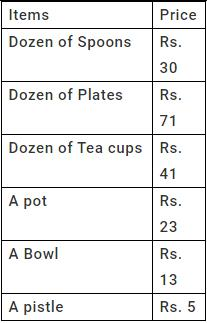Q. How much is a pot more expensive than a bowl?

Solution:
QUESTION: 15

Directions: for questions: See the table blow and answer the following questions: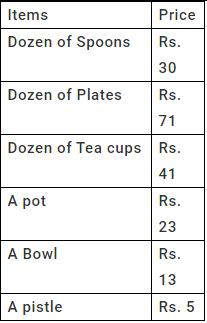Q. What is the total cost of items on the price list?

Solution:
QUESTION: 16

Lorain bought a packet of peanuts for Rs. 13. If she paid Rs. 1,000, how much change did she receive?

Solution:
QUESTION: 17

Nicole parked her car at a parking for 2 hours in which 1 hour costs Rs. 1. On paying she gave Rs. 50. How much change did she receive?

Solution:
QUESTION: 18

Fire crackers cost Rs. 20 and a Christmas tree costs Rs. 53. If I pay Rs. 100 for a fire cracker and an Xmas tree, how much will my balance be?

Solution:
QUESTION: 19

A day at the hotel costs Rs. 100. If I spend 10 days and pay Rs. 1,000 at the reception, how much is my change going to be?

Solution:

Because if cost of 1 day =100

cost of 10 days =100*10

=1000

so 1000 rupee you have to pay

QUESTION: 20

John had Rs. 500 to buy drinks and sandwiches for his birthday party. He bought 5 small bottles of drinks at Rs. 24 per bottle and 8 sandwiches at Rs. 16 per piece. How much money was left after the shopping?

Solution: